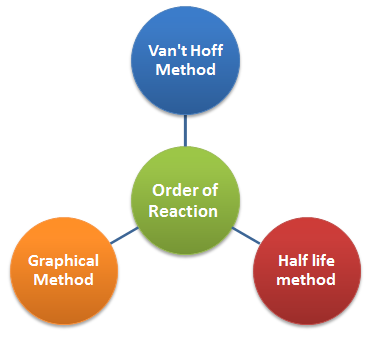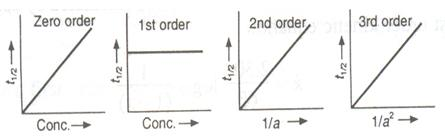Click to Chat

1800-1023-196

+91-120-4616500

CART 0

• 0

MY CART (5)

Use Coupon: CART20 and get 20% off on all online Study Material

ITEM
DETAILS
MRP
DISCOUNT
FINAL PRICE
Total Price: Rs.

There are no items in this cart.
Continue ShoppingMethods for Determination of Order of a Reaction

Table of Content

Initial Rate Method

Graphical Method

Half Life Method

Van't Hoff Differential Method

Related ResourcesInitial Rate MethodIn this method initial rate of reaction is determined by varying the concentration of one of the reactants while others are kept constant

R = k[A]x[B]y[C]z

if [B] & [C] = Constant
then for two different initial concentrations of A we have

and

Graphical Method

This method can be used when there is only one reactant.

If the plot of log [A] vs t is a straight line, the reaction follows first-order .

If the plot of 1/[A] vs t is a straight line, the reaction follows second order.

If the plot of 1/[A]2 is a straight line , the reaction follows third order.

Generally, for a reaction of nth order, a graph of 1/[A]n-1 vs t must be a straight line.

Here [A] is the concentration of reactant at any given time of the reaction (other t =0). [A] = (a-x) where a is the initial concentration  and x is the extent of reaction at time t.

Refer to the following video for Determination of Order of a Reaction

Half Life Method

This method is used only when the rate law involved by only one concentration term.

t(1/2)  ∞ a1-n

t(1/2) = k’ 1/an-1

log t(1/2) = log k’ + (1-n)a

Graph of logt1/2 vs log a, gives a straight line with slope (1-n) , where 'n' is the order of the reaction.

Determining the slope we can find the order n.

If half life at different concentrations is given then.

and

Taking logarithm and rearranging

Plots of half-lives concentration (t1/2  ∞  a1-a):This relation can be used to determine order of reaction 'n'

Van't Hoff Differential Method

As we know that, the rate of a reaction varies as the nth power of the concentration of the reactant where 'n' is the order of the reaction.

Thus, for two different initial concentrations C1 and C2, equations can be written in the form

….(i)

and

....(ii)

Taking logarithms,

Subtracting Eq. (ii) from (i),

or

n = [log(-(dC1)/dt)-log((dC2)/dt)] ÷ [logC1 - log C2]     ....(iii)

-dc1/dt and -dc2/dt are determined from concentration vs. time graphs and the value of 'n' can be determined.

Solved Example

Question:

From the following data show that the decomposition of hydrogen peroxide in aqueous solution is a first - order reaction. What is the value of the rate constant?

Time in minutes

0

10

20

30

40

Volume V in ml

25.0

20.0

15.7

12.5

9.6

where V is the number of ml of potassium permanganate required to decompose a definite volume of hydrogen peroxide solution.

Solution:

The equation for a first order reaction is

The volume of KMnO4 used, evidently corresponds to the undecomposed hydrogen peroxide.

Hence the volume of KMnO4 used, at zero time corresponds to the initial concentration a and the volume used after time t, corresponds to (a - x) at that time. Inserting these values in the above equation, we get

when t = 10 min. k1 = 2.23/10 log (25/20) = 0.022318 min-1 = 0.000372 s-1

when t = 20 min. k1 = 2.23/20 log (25/12) = 0.023265 min-1 = 0.0003871 s-1

when t = 30 min. k1 = 2.23/30 log (25/12.5) = 0.02311 min-1 = 0.000385 s-1

when t = 40 min. k1 = 2.23/40 log (25/19.6) = 0.023932 min-1 = 0.0003983 s-1

The constancy of k, shows that the decomposition of H2O2 in aqueous solution is a first order reaction.

The average value of the rate constant is 0.0003879 s-1.Question 1:

In which of the following methods initial rate of reaction is determined by varying the concentration of one of the reactants while others are kept constant

a. Graphical method

b. Initial rate method

c. Half life method

d. Van’t hoff method

Question 2:

If the plot of 1/[A]2 is a straight line , the reaction follows

a. zero order

b. first order

c. second order

d. third order

Question 3:

log t(1/2) =

a. log k’ - (1-n)a

b. log k’ + (1-n)a

c. -log k’ + (1-n)a

d. log k’ / (1-n)a

Question 4:

Which of the following methods is used only when the rate law involved by only one concentration term?

a. Graphical method

b. Initial rate method

c. Half life method

d. Van’t hoff methodQ.1
Q.2
Q.3
Q.4

b
d
b
c

Related Resources

Refer to the Books of Chemistry

To read more, Buy study materials of Chemical Kinetics comprising study notes, revision notes, video lectures, previous year solved questions etc. Also browse for more study materials on Chemistry here.### Course Features

• 731 Video Lectures
• Revision Notes
• Previous Year Papers
• Mind Map
• Study Planner
• NCERT Solutions
• Discussion Forum
• Test paper with Video Solution